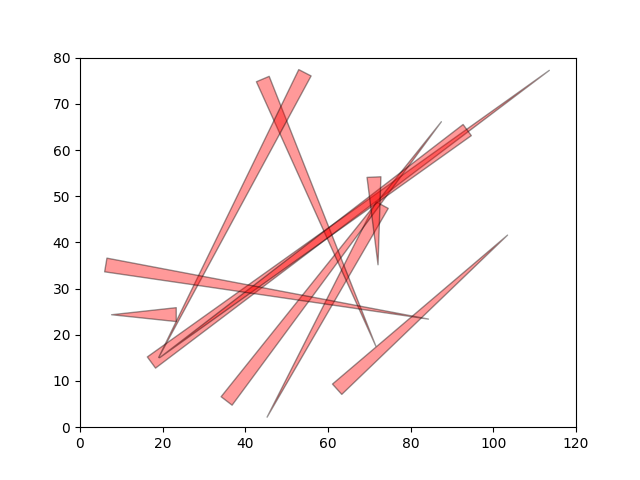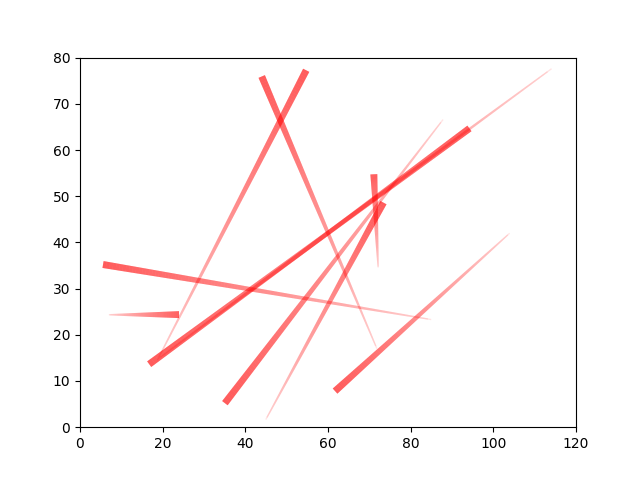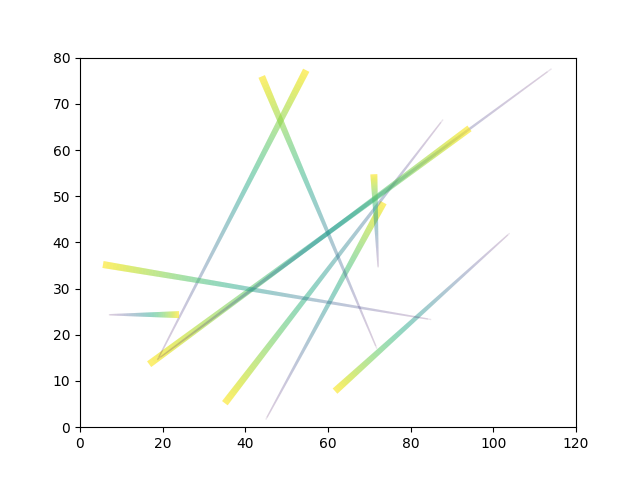# Plot wedges

This example shows how you can plot wedge and comet lines on normal axes.

```import matplotlib.pyplot as plt
import numpy as np
```

## Generate random data

First let’s generate some random data

```np.random.seed(42)
x = np.random.uniform(0, 120, 10)
y = np.random.uniform(0, 80, 10)
x_end = np.random.uniform(0, 120, 10)
y_end = np.random.uniform(0, 80, 10)
```

## Plot using wedges

This shows how to plot the lines using wedges. The code was donated by Jon Ollington

```fig, ax = plt.subplots()
ax.set_xlim(0, 120)
ax.set_ylim(0, 80)
for i in range(len(x)):
ax.annotate('', xy=(x[i], y[i]), xytext=(x_end[i], y_end[i]), zorder=2,
arrowprops=dict(arrowstyle="wedge,tail_width=1", linewidth=1,
fc='red', ec='black', alpha=0.4),
)
```## Plot using lines

You can also use mplsoccer’s lines function on normal axes.

```from mplsoccer import lines

fig, ax = plt.subplots()
ax.set_xlim(0, 120)
ax.set_ylim(0, 80)
lc1 = lines(x, y, x_end, y_end, color='red', comet=True, transparent=True,
alpha_start=0.1, alpha_end=0.4, ax=ax)
```## Plot using cmap

You can also use plot using a color map using mplsoccer’s lines function.

```fig, ax = plt.subplots()
ax.set_xlim(0, 120)
ax.set_ylim(0, 80)
lc2 = lines(x, y, x_end, y_end, cmap='viridis', comet=True, transparent=True,
alpha_start=0.1, alpha_end=0.4, ax=ax)

plt.show()  # If you are using a Jupyter notebook you do not need this line
``````/home/docs/checkouts/readthedocs.org/user_builds/mplsoccer/checkouts/latest/mplsoccer/cm.py:57: MatplotlibDeprecationWarning: The get_cmap function was deprecated in Matplotlib 3.7 and will be removed two minor releases later. Use ``matplotlib.colormaps[name]`` or ``matplotlib.colormaps.get_cmap(obj)`` instead.
cmap = get_cmap(cmap)
```

Total running time of the script: ( 0 minutes 0.397 seconds)

Gallery generated by Sphinx-Gallery